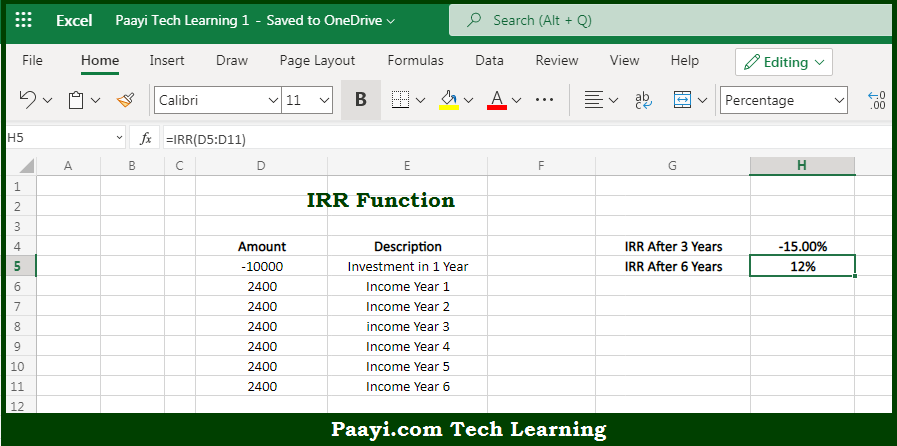# Learn How to Use Microsoft Excel IRR Function

Written by | 0 Comments | 435 Views

In this article, you will learn how to use the Microsoft Excel IRR function and its prime function in Microsoft Excel. You will also get to know the Microsoft Excel IRR function return value and syntax with the help of some examples.

Microsoft Excel IRR Function

The main purpose of the Microsoft Excel IRR function is to calculate internal rate of return. That implies, with the help of IRR function you can able to return the internal rate of return (IRR) for a series of cash flows that occur at regular intervals. So, with the help of IRR function, you can able to calculate the internal rate of return.

Return Value of IRR Function

The return value will be the calculated return as a percentage.

Syntax of IRR Function

=IRR(values, [guess])

Where the arguments:

values: This is the array or reference to cells that contain values.

guess: This is an estimate for the expected IRR. Default is .1 (10%) (optional).

## How to Use Microsoft Excel IRR Function?So we know that Microsoft Excel IRR function you can able to calculate the internal rate of return. That implies, with the help of the IRR function you can able to return the internal rate of return (IRR) for a series of cash flows that occur at regular intervals. So, with the help of the IRR function, you can able to calculate the internal rate of return.